HiSET Math Practice Test

Try our free HiSET Math practice test. The HiSET Math test has 50 multiple-choice questions that must be answered within 90 minutes. Topics covered include numerical operations, measurement, geometry, data analysis, probability, statistics, and algebra. A calculator is not required, but the test center will have calculators available. A formula sheet will also be provided. Start your test prep right now with our HiSET Math practice test!

Congratulations - you have completed . You scored %%SCORE%% out of %%TOTAL%%. Your performance has been rated as %%RATING%%
 Question 1
Kayla owns a house cleaning company and must give price quotes to potential customers. She determines her price by assuming a \$25 base charge and then adding \$8 for each bathroom and \$4 for every other room. If she uses P to represent the price, B to represent the bathrooms, and R to represent the other rooms, which of the following defines her price quote formula?

 A P = 25 + 12(BR) B P = 25(4R + 8B) C P = 25 + 8B + 4R D P = (4)(8)(R + B) + 25 E P = (25 + 8B) + (25 + 4R)
Question 1 Explanation:
The correct answer is (C). The price quote, P, will be equal to the total amount of charges. It’s given that there is a \$25 base charge, so we can begin by writing the price quote as P = \$25. To this base charge of \$25, \$8 per each bathroom, represented by the variable B, is added; our price quote is now P = \$25 + \$8B. Lastly, \$4 per each other room, R, is added, and the actual price quote is P = \$25 + \$8B + \$4R, or P = 25 + 8B + 4R.
 Question 2
If a car travels 360 kilometers in 5 hours, how many kilometers will it travel in 9 hours when driving at the same speed?

 A 72 B 288 C 620 D 648 E 720
Question 2 Explanation:
The correct answer is (D). First calculate the distance the car travels in 1 hour:

360 km ÷ 5 hours = 72 km per hour

Then multiply this number by the total number of hours traveled:

9 hours × 72 km per hour = 648 km

Notice that the final calculation yields the correct units; this helps to confirm that the calculation is correct.
 Question 3
Aisha wants to paint the walls of a room. She knows that each can of paint contains one gallon. A half gallon will completely cover a 55 square feet of wall. Each of the four walls of the room is 10 feet high. Two of the walls are 10 feet wide and two of the walls are 15 feet wide. How many 1-gallon buckets of paint does Aisha need to buy in order to fully paint the room?

 A 4 B 5 C 9 D 10 E 15
Question 3 Explanation:
The correct answer is (B). The total number of buckets necessary will be the total area of the walls divided by the total area covered by each bucket. First, calculate the area of the walls Aisha wants to paint. Two of the walls are 10 × 10 and two of the walls are 10 × 15:

2 (10 × 10) = 200 sq. ft.
2 (10 × 15) = 300 sq. ft.
So the total square footage of the walls is 500.

If a half gallon of paint will cover 55 square feet, then each gallon will cover 2 × 55 = 110 square feet. Four gallons can only cover 440 square feet. Five gallons will cover 550 square feet, which will be enough for the entire area of the walls.
 Question 4
There are 4 red marbles and 8 green marbles in a box. If Emma randomly selects a marble from the box, what is the probability of her selecting a red marble?

 ABCD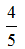E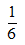Question 4 Explanation:
The correct answer is (A). The probability of a successful event is the ratio of the number of successful events to the total number of events. In this case, a successful event is selecting a red marble from the box. The total number of events is the total number of marbles in the box: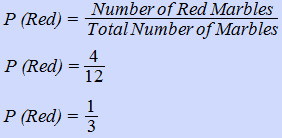Question 5
If Maria left a \$10.16 tip on a breakfast that cost \$86.89, approximately what percentage was the tip?

 A 12% B 13% C 17% D 25% E 31%
Question 5 Explanation:
The correct answer is (A). If you want to know what percent A is of B, you divide A by B, then multiply that number by 100%:

10.16 ÷ 86.89 = 0.1169 × 100% = 11.69%

This is approximately 12%.
 Question 6
Sofía's Restaurant offers the following choices:If the only dinner you can order must include one salad, one main course, and one dessert, how many different combinations of this dinner are possible?

 A 12 B 60 C 120 D 144 E 360
Question 6 Explanation:
The correct answer is (B). This can be solved with the Counting Principle: If there are m ways for one activity to occur and n ways for a second activity to occur, then there are m × n ways for both to occur.

To solve this problem, multiply 4 (the number of salads) × 5 (the number of main courses) × 3 (the number of desserts):

4 × 5 × 3 = 60 different combinations
 Question 7
Factor: 4y(3x + 2) − 2(3x + 2)

 A (4y − 2)(3x + 2) B (3x − 2)(4y + 2) C (4y + 2)(6x) D (3x + 2)(4y + 2) E (4y − 6)(12x + 2)
Question 7 Explanation:
The correct answer is (A). Any part of an expression that is common to every term in the expression can be factored out. In this case, (3x + 2) is a common term and can be factored out from both parts of the expression:

4y(3x + 2) − 2(3x + 2) = (3x + 2)[4y − 2]

Notice that distributing (3x + 2) through [4y − 2] yields the original expression; this verifies the answer.
 Question 8
Five years ago, Amy was three times as old as Mike. If Mike is 10 years old now, how old is Amy?

 A 15 B 20 C 25 D 30 E 35
Question 8 Explanation:
The correct answer is (B). Set up a table comparing the situation five years ago to the situation today to keep things organized.Mike is 10 years old now, so he must have been 5 years old five years ago.

Amy was 3 times as old as Mike five years ago, so Amy must have been 15 five years ago.

Add 5 years to Amy’s age five years ago to calculate her age now. Amy is 15 + 5 = 20 years old now.
 Question 9
Simplify:AB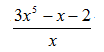CD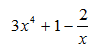E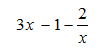Question 9 Explanation:
The correct answer is (C). Completely simplify the expression by dividing every term in the numerator by the term in the denominator: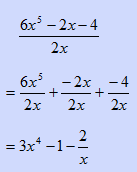Question 10
Mason earns \$8.10 per hour and worked 40 hours. Noah earns \$10.80 per hour. How many hours would Noah need to work to equal Mason’s earnings over 40 hours?

 A 15 B 25 C 27 D 28 E 30
Question 10 Explanation:
The correct answer is (E). Begin by calculating Mason’s total earnings after 40 hours:

40 hours × \$8.10 per hour = \$324

Next, divide this total by Noah’s hourly rate to find the number of hours Noah would need to work:

\$324 ÷ \$10.80 per hour = 30 hours
 Question 11
The area of the circle shown below is 100π. What is the diameter (D) of the circle?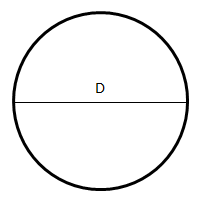A 10 B 20 C 30 D 40 E 50
Question 11 Explanation:
The correct answer is (B). Begin by writing out the formula for the area of a circle, which is one of the formulas provided on the Formula Sheet. Next, substitute the given value for the area, and then solve for the radius. Recall that the square root of both sides must be evaluated in order to isolate r. Multiplying the value of r by 2 gives the diameter: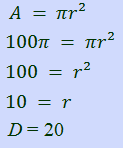Question 12
Emma has a budget of \$300 for her holiday spending. She decides to buy a pair of shoes for each of her grandchildren. If each pair of shoes costs c dollars, and Emma has 7 grandchildren, which of the following inequalities represents her budget constraint?

 A 7c > 300 B c + 7 > 300 C 7 + c ≤ 300 D 7c ≤ 300 E 7 > 300c
Question 12 Explanation:
The correct answer is (D). If Emma buys 7 pairs of shoes at a cost of \$c each, she pays 7c. Since she only has \$300 to spend, 7c must be less than or equal to \$300:

7c ≤ 300
 Question 13
There are 5 blue marbles, 4 red marbles, and 3 yellow marbles in a box. If Isabella randomly selects a marble from the box, what is the probability of her selecting a red or yellow marble?

 A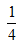B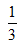C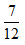DEQuestion 13 Explanation:
The correct answer is (C). A probability is the likelihood of a successful event occurring divided by the total number of events possible. In this case, a successful event is selecting either a red or a yellow marble and the total number of events possible is the total number of marbles.

Number of red and yellow marbles: 4 + 3 = 7

Total number of marbles: 5 + 4 + 3 = 12

Probability: 7/12
 Question 14
There are two pizza ovens in a restaurant. Oven #1 burns three times as many pizzas as Oven #2. If the restaurant had a total of 12 burnt pizzas on Saturday, how many pizzas did Oven #2 burn?

 A 2 B 3 C 4 D 5 E 6
Question 14 Explanation:
The correct answer is (B). Begin by expressing the relationship between the ovens with an equation. Use a variable to represent the number of burnt pizzas. For every x pizzas burnt by Oven #2, Oven #1 burns 3x pizzas. It is given that 12 total pizzas are burnt, and the total number of burnt pizzas is a combination of pizzas burnt by Oven #1 and Oven #2:

x + 3x = 12
4x = 12
x = 3

The variable x represents the number of pizzas burnt by Oven #2, so answer choice (B) is correct.
 Question 15
Simplify:

(x6)(x5)

 A 2x11 B 2x30 C x1 D x30 E x11
Question 15 Explanation:
The correct answer is (E). Recall that the multiplication of terms sharing the same base can be simplified by adding the exponents:

xa * xb = x(a + b).

If this rule is forgotten, you can quickly derive it by considering the simple case of 21 * 22, which can be rewritten as 2 * 2 * 2, or 23. The expression in this case, then, can be simplified as:

(x6) (x5) = x5+6
= x11
 Question 16
Each player on a high school football team has been weighed, and the data are shown in the box-and-whisker plot below.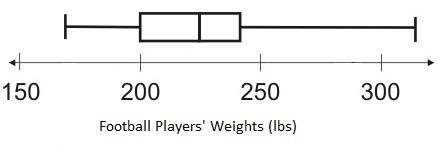Based on this box-and-whisker plot, which of the following statements is accurate?

 A The heaviest player weighs 300 lbs. B One-fourth of the players weigh less than 200 lbs. C The median player weighs 245 lbs. D The lightest player weighs 200 lbs. E The average player is heavier this year than last year.
Question 16 Explanation:
The correct answer is (B). The ability to interpret data from a box-and-whisker plot is a required skill for the HiSET Math test. Here is the meaning of each part of the box-and-whisker plot: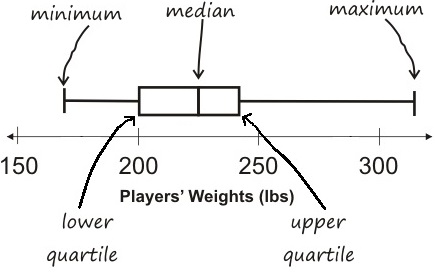In this example, the minimum value represents the lightest player on the team, and the maximum value represents the heaviest player on the team. The median value is the player who is at the midpoint of the distribution. The quartile values divide the distribution into fourths. Since the lower quartile is at 200, it is accurate to say that one-fourth of the players weigh less than 200 lbs.
 Question 17
If y = 3ab + 2, what is y when a = 1 and b = 2?

 A 16 B 18 C 20 D 22 E 32
Question 17 Explanation:
The correct answer is (D). Substitute the given values for a and b into the equation and then evaluate to find y:

y = 3ab + 2b³
y = 3(1)(2) + 2(2)³
y = 6 + 16
y = 22
 Question 18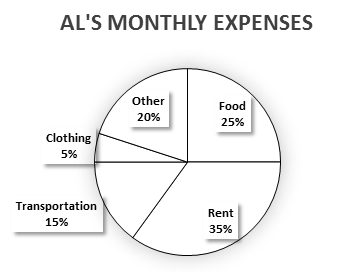This pie chart shows Al’s monthly expenses. If Al spent a total of \$1,550 in one month, how much did he spend on clothes in that month?

 A \$77.50 B \$232.50 C \$775 D \$7.75 E \$1,627.50
Question 18 Explanation:
The correct answer is (A). Remember that 5% is equivalent to 5/100 or .05:

.05 × \$1,550 = \$77.50
 Question 19This pie chart shows Al’s monthly expenses. If Al spent \$210 on transportation in one month, how much did he spend on food in that month?

 A \$126 B \$250 C \$325 D \$350 E \$525
Question 19 Explanation:
The correct answer is (D). One method for solving this problem is to recognize that the ratio of the amount Al spends on food to the amount he spends on transportation will equal the ratio of the percentage of his income spent on food to the percentage spent on transportation. Expressed mathematically: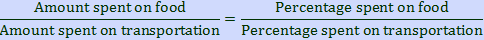Substitute the known values to then solve for the unknown amount spent on food: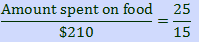Cross multiply and simplify to solve: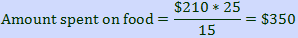Question 20
A toy manufacturer makes 15,000 toys per year. The company randomly selects 300 of the toys to sample for inspection. The company discovers that there are 5 faulty toys in the sample. Based on the sample, how many of the 15,000 total toys are likely to be faulty?

 A 25 B 250 C 350 D 625 E 650
Question 20 Explanation:
The correct answer is (B). The sample indicates that 5 out of every 300 randomly selected toys will be faulty. Consequently, a proportion can be set up that relates the unknown number of faulty toys in the total number of toys to the ratio of faulty toys to the sample. Stated otherwise:T is the unknown number of faulty toys in the total. Multiply both sides by 15,000 and then divide the left-hand side by 300 to solve for T:Question 21
What is the slope of the line that passes through points (0,5) and (7,0) on the coordinate plane shown below?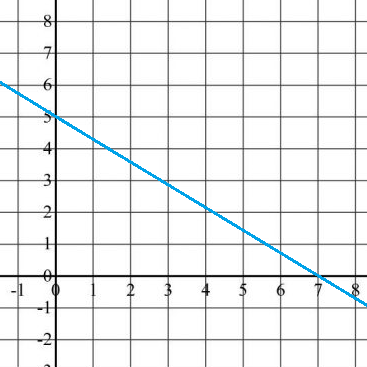A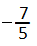BCDEQuestion 21 Explanation:
The correct answer is (B). The slope of a line, which can be remembered as “the rise over the run,” is the change in y values divided by the change in x values across two points on a line. Written algebraically, the slope is defined as: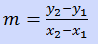Substitute the given points (0,5) and (7,0) into the equation to calculate the slope:Question 22
Which of the following expressions is equivalent to 3x(2 + 5y)?

 A 3x + 5y B 6x + 5y C 6x + 15y D 6x + 15xy E 3x + 5y + 2
Question 22 Explanation:
The correct answer is (D). Begin by noticing the form in which the answer choices are written. As no answer choice contains a parenthetical expression, start by distributing the 3x through the parentheses. Multiply 3x by each of the terms inside of the parentheses and combine any like terms:

3x(2 + 5y)
= 3x(2) + 3x(5y)
= 6x + 15xy
 Question 23
Max struggled with his math class early in the year, but he has been working hard to improve his scores. There is one test left, and he is hoping that his final average test score will be 75. What score will he need to get on Test 6 to finish the year with an average score of 75?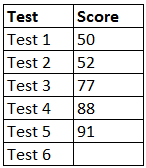A 75 B 85 C 92 D 98 E 100
Question 23 Explanation:
The correct answer is (C). The average of a set of data points is the sum of the data points divided by the total number of data points. In this case, we are given 5 out of 6 data points, the number of data points, and the desired average. Substitute the given values into the formula for the average, using a variable to represent the unknown test score, and then solve for the variable:

Average = sum of data points ÷ number of data points

75 = (50 + 52 + 77 + 88 + 91 + x) ÷ 6

75 = (358 + x) ÷ 6

Next, eliminate the denominator by multiplying both sides by 6:
450 = 358 + x

The last step is to subtract 358 from both sides:
x = 92
 Question 24
(3x + 4y)(2x + 5y) = ?

 A 3x² + 8xy + 20y² B 3x² + 15xy + 20y² C 6x² + 15xy + 20y² D 6x² + 23xy + 20y² E 3x² + 23xy + 9y²
Question 24 Explanation:
The correct answer is (D). The “FOIL method” is the easiest way to remember how to multiply expressions of the form (x + y)(a + b). Multiply the First two terms, then the Outer two terms, then the Inner two terms, and then the Last two terms, then sum all four to arrive at the answer:

(3x + 4y)(2x + 5y)
6 + 15xy + 8xy + 20
6 + 23xy + 20
 Question 25
What is 181.5% of 18?

 A 3.267 B 14.67 C 32.67 D 3267 E 18.15
Question 25 Explanation:
The correct answer is (C). The key word ‘of,’ indicates multiplication of the percentage with the value. First, convert the fraction to a decimal by moving the decimal point 2 places to the left to give 1.815. Then multiply this by 18.

1.815 × 18 = 32.67
 Question 26
Solve for x:

3(x + 1) = 5(x − 2) + 7

 A −2 B 2 C ½ D −3 E 3
Question 26 Explanation:
The correct answer is (E). Begin by distributing the 3 and the 5 through their respective parentheses, then combine like terms on each side of the equal sign:
3(x + 1) = 5(x − 2) + 7
3x + 3 = 5x − 10 + 7
3x + 3 = 5x − 3

Add 3 to both sides to maintain the equality:
3x + 6 = 5x

Subtract 3x from both sides and then divide the resulting equation by 2 to solve for x as follows:
6 = 2x
3 = x

Remember that any time a variable is solved for in an equation, the calculated value can be verified by substituting it into the original equation and determining whether it yields a true statement.
 Question 27
Consider the list:

2, 2, 3, 5, 9, 11, 17, 21

If the number 23 is added to the list, which measurement will NOT change?

 A Mean B Median C Mode D Range E Average
Question 27 Explanation:
The correct answer is (C). The mode will not change. The mode is the number that appears the most frequently; in this case it is 2. The remaining measures will change:

The mean is the average, and in this case, it will increase because a number larger than the current average is added to the list.

The median is the number in the middle, and it will change from 7 to 9.

The range is the difference between the highest and lowest numbers and will change from 19 to 21 because a new maximum value is added to the list (21 − 2 = 19 and 23 − 2 = 21).
 Question 28
Calculate the value of x for the right triangle shown below.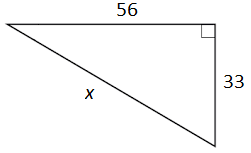A 66 B 89 C 56 D 65 E 75
Question 28 Explanation:
The correct answer is (D). The Pythagorean theorem can be used to find a missing segment of a right triangle if two side lengths are given. The theorem relates the legs of a right triangle to its hypotenuse as a2+ b2 = c2, where a and b are the legs and c is the hypotenuse. The hypotenuse is the longest side in a right triangle and is always opposite the right angle.

Substitute the known values into their appropriate places and solve for the unknown side length:
332 + 562 = c2

Evaluate the squares:
1,089 + 3,136 = c2
4,225 = c2

Evaluate the square root of both sides: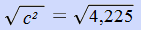c = 65
 Question 29
List A consists of the numbers {2, 9, 5, 1, 13}, and list B consists of the numbers {7, 4, 12, 15, 18}.

If the two lists are combined, what is the median of the combined list?

 A 5 B 6 C 7 D 8 E 9
Question 29 Explanation:
The correct answer is (D). Recall that the median of a set of data is the value located in the middle of the data set. In the case of a data set that contains an even number of numbers, the median is the average of the two middle numbers. Combine the 2 sets provided, and organize them in ascending order:

{1, 2, 4, 5, 7, 9, 12, 13, 15, 18}

Since there are an even number of items in the resulting list, the median is the average of the two middle numbers.

Median = (7 + 9) ÷ 2 = 8
 Question 30
In a coordinate plane, triangle ABC has coordinates: (−2,7), (−3,6), and (4,5). If triangle ABC is reflected over the y-axis, what are the coordinates of the new image?

 A (−2,−7), (−3,−6), (−4,−5) B (−2,−7), (−3,−6), (4,−5) C (2,7), (3,6), (−4,5) D (2,7), (3,6), (4,5) E (7,−2), (6,−3), (5,4)
Question 30 Explanation:
The correct answer is (C). One way to solve this problem is to draw the figure and then count how many units each point is from the y-axis, and to then count that many units in the opposite direction. A more efficient method is to recognize that reflecting over the y-axis causes the x-value to switch sign, but does not influence the y-value of the point.

According to the second method, reflecting (−2,7), (−3,6), (4,5) across the y-axis produces the points (2,7), (3,6), (−4,5).
 Question 31
If the height of a right pyramid is 6 cm and its base is a square with side 2 cm, what is its volume?

 A 6 cm³ B 8 cm³ C 12 cm³ D 24 cm³ E 36 cm³
Question 31 Explanation:
The correct answer is (B). If you don't remember the formula for the volume of a pyramid, you can find it on the HiSET Formula Sheet that is provided:

Volume = 1/3(area of the base)(height)

Plug the values into the formula and calculate. Since the base is a square with side lengths of 2, you will need to multiply 2 × 2 to find the area of the base.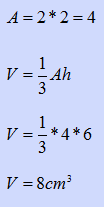Question 32
A river rafting guide offers group trips. She charges \$250 for 2 people and \$40 more for each additional person. If 5 friends share the cost of a trip equally, how much will each person pay?

 A \$40 B \$58 C \$74 D \$90 E \$370
Question 32 Explanation:
The correct answer is (C). First calculate the entire cost of the trip. Start with \$250 for the first two people and then add \$40 for each of the other three:

250 + 40 + 40 + 40 = \$370

Then divide this amount by 5 to determine the amount that each person will pay:

\$370 ÷ 5 = \$74
 Question 33
Multiply and write the product in scientific notation:

(7.9 × 105) × (4.1 × 10−3)

 A 3.239 × 101 B 3.239 × 102 C 3.239 × 104 D 3.239 × 10−15 E 3.239 × 103
Question 33 Explanation:
The correct answer is (E). To multiply two numbers in scientific notation, multiply their coefficients and add together the exponents of the base 10:

(7.9 × 105) × (4.1 × 10−3)

= (7.9 × 4.1) × (105 × 10−3)
= (32.39) × (105−3)
= (32.39) × (102)

When writing scientific notation, the absolute value of the coefficient should be at least one but less than ten:

= (3.239) × (103)
 Question 34
Diego’s current age is five times Martina’s age ten years ago. If Martina is currently m years old, what is Diego’s current age in terms of m?

 A 5m B 5m − 10 C 5m − 50 D 5m + (m − 10) E 10(m − 5)
Question 34 Explanation:
The correct answer is (C). Martina’s age ten years ago is:
m − 10

So Diego’s age is:
5(m − 10)
= 5m − 50
 Question 35
The points represented by the (x, y) coordinate pairs in the table below all lie on line k.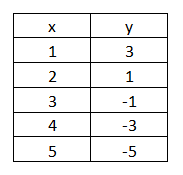What is the slope of line k?

 A −5 B −3 C −2 D 2 E 5
Question 35 Explanation:
The correct answer is (C). We are told that all the points lie on line k. Therefore, we can calculate the slope as the change in y divided by the change in x using any two of the points. Using points (1, 3) and (5, −5) the slope is calculated as follows: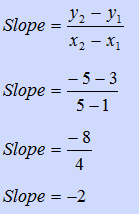Question 36
Liam is driving to Utah. He travels at 70 kilometers per hour for 2 hours, and 63 kilometers per hour for 5 hours. Over the 7 hour time period what was Liam's average speed?

 A 64 km/h B 65 km/h C 66 km/h D 67 km/h E 68 km/h
Question 36 Explanation:
The correct answer is (B). To find the average, use the following formula:

Average Speed = Total Distance ÷ Total Time

Total Distance = 2 hours × 70 km/h + 5 hours × 63 km/h
= 140 km + 315 km
= 455 km

Total Time = 7 hours

Average Speed = 455 km ÷ 7 hours
= 65 km/h
 Question 37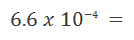A 6.6e-05 B 0.00066 C 0.0066 D 0.066 E 0.66
Question 37 Explanation:
The correct answer is (B). Multiplying a decimal value by 10 raised to a power is equivalent to moving the decimal point to the left or right the number of times indicated by the power.

In the case of a negative exponent, the decimal is moved to the left (this is the same as dividing by 10 a number of times).

In the case of a positive exponent, the decimal is moved to the right (this is the same as multiplying by 10 a number of times).

The negative exponent here, −4, indicates that the decimal point is to be moved to the left 4 places:Question 38
Karen, James, Frank, and Dan were left an inheritance by their grandfather. If Karen receives one-eighth of the inheritance, James receives one quarter of the inheritance, Frank receives three-eighths of the inheritance, and Dan receives the remainder, what fraction of the inheritance does Dan receive?

 AB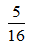C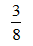DEQuestion 38 Explanation:
The correct answer is (A). We can add up the fractions received by Karen, James, and Frank as follows:We can find the fraction Dan receives by subtracting the sum of the other three fractions from one, since all the fractions must add up to one. Dan will receive: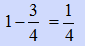Question 39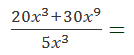A 6 + 6x6 B 4 + 6x6 C 4x3 + 6x9 D 4x3 + 6x3 E 4 + 6x3
Question 39 Explanation:
The correct answer is (B). Begin every problem involving polynomials in the numerator or denominator by considering the factoring options. Here, the numerator can be factored, as there is a coefficient and variable common to both terms: 10x3. However, before factoring out the full coefficient, notice that dividing out the full 10 would entail multiplying the resulting 2 back through the numerator. Factor out 5x3 and divide by the denominator to arrive at the answer: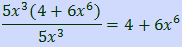Question 40
The data in the table below shows the results of Tracy trying to train her dog Snowy.In what percentage of these trials did Snowy obey the command given?

 A 25% B 37.5% C 50% D 62.5% E 75%
Question 40 Explanation:
The correct answer is (C). Calculate the percentage of trials that Snowy obeyed by dividing the number of times Snowy obeyed by the total number of commands given. The number of times Snowy obeyed: trial 1, trial 3, trial 6, trial 8, which sums to 4 trials. There are 8 total trials so the percentage is:Question 41
Which of the following is a rational number?

 A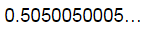B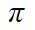C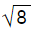D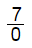E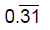Question 41 Explanation:
The correct answer is (E). A rational number is a number that can be expressed as a fraction with a denominator that is not zero.

Non-terminating, non-repeating decimals are irrational numbers. This would include 0.5050050005..., π (3.14159....), and √8 (2.82842...). Answer (D) is irrational because dividing by zero is undefined.

Answer (E) is a repeating decimal. Terminating decimals and repeating decimals are rational because they can be written as fractions.
 Question 42
In the figure below, lines d and f are parallel and the angle measures are as given. What is the value of x?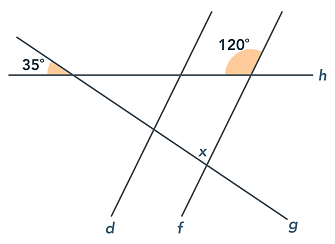A 55 B 75 C 85 D 90 E 120
Question 42 Explanation:
The correct answer is (C). Vertical angles are the angles opposite of each other that are created when two lines cross. Vertical angles are always equal. So, the angle vertical to the 35-degree angle also has a measure of 35 degrees. The supplement of the 120-degree angle has a measure of 60 degrees (all straight lines measure 180 degrees), so we then have a triangle with angles measuring 35, 60, and x degrees, as shown in the figure provided. Since the angles of a triangle add to 180 degrees:

35 + 60 + x = 180
x = 180 − 35 − 60
x = 85 degrees
 Question 43
Valentina purchased a new hat that was on sale for \$5.06. The original price was \$9.20. What percentage discount was the sale price?

 A 4.5% B 41.4% C 45% D 55% E 55.5%
Question 43 Explanation:
The correct answer is (C). The percentage discount is the reduction in price divided by the original price. The difference between original price and sale price is:

\$9.20 − \$5.06 = \$4.14

The percentage discount is this difference divided by the original price:

\$4.14 ÷ \$9.20 = 0.45

Convert the decimal to a percentage by multiplying by 100%:

0.45 × 100% = 45%
 Question 44
ABC is a triangle with coordinates (3, 1), (6, 1), and (1, 3). It is translated to points A′(−3, 3), B′( 0, 3), and C′(−5, 5). Find the rule for the translation.

 A 6 units right and 2 units down B 2 units left and 6 units up C 6 units down and 2 units right D 6 units left and 2 units up E 2 units down and 2 units right
Question 44 Explanation:
The correct answer is (D). The rule of translation is the rule, which when applied to the first set of points, yields the second set of points. The difference between the x values of the non-translated and translated points is:

(x2x1) = (−3 − 3) = (0 − 6) = (−5 − 1) = −6

This corresponds to a shift of 6 units to the left.

Likewise, for the y values:

(y2y1) = (3 − 1) = (3 − 1) = (5 − 3) = 2

This corresponds to a vertical shift of 2 units.
 Question 45
Anastasia needs to order liquid fertilizer for her landscaping company. She plans to keep the fertilizer in a large cylindrical storage tank, but isn’t sure how much it will hold. The tank is 10 feet tall and the circular base has a diameter of 10 feet. What is the volume of her storage tank?

 A 78.5 ft³ B 157 ft³ C 3,140 ft³ D 1,570 ft³ E 785 ft³
Question 45 Explanation:
The correct answer is (E). In cases where you are unsure of the correct formula to use, be sure to check the formula sheet that is provided for HiSET math questions. The formula for a cylinder is one of the provided formulas:

Volume = (area of the base) (height)

The base of a cylinder is a circle, so you will also need the formula for the area of a circle, which is also provided on the formula sheet:

Combining these formulas, we get:

V = πr²h

V is volume, r is the radius, and h is the height of the cylinder. (Remember that the radius of a circle is half of the diameter.) Substitute the given values into the equation to solve for the volume:
V = π * (5)² * 10
V = 250π

Recall that π (pi) is approximately equal to 3.14:
250 * 3.14 = 785
 Question 46
Each day, Deion takes two pills of type A medicine, three pills of type B medicine, and one pill of type C medicine.  How many pills will he take in 14 days if he forgets to take the type B pills on each of two days?

 A 84 B 81 C 78 D 75 E 72
Question 46 Explanation:
The correct answer is (C). Each day, Deion is supposed to take a total of six pills. If he takes all of his medication for 14 days, he will take (14)(6) = 84 pills. If he forgets to take the three pills of type B on two occasions, this total will reduce by (3)(2) = 6 pills. If he forgets to take his type B pills, he will take 84 – 6 = 78 pills in 14 days.
 Question 47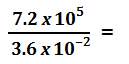A 2.0 x 10−14 B 2.0 x 10−3 C 2.0 x 103 D 3.6 x 103 E 2.0 x 107
Question 47 Explanation:
The correct answer is (E). Begin by expressing the fraction as the product of two fractions: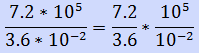Recall that division of exponents sharing the same base can be rewritten as a base raised to the difference of the exponents: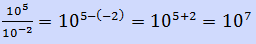So, the expression can be rewritten as: 2.0 x 107
 Question 48
Three boxes of crayons, identified as Box A, Box B, and Box C, contain the same four colors, as shown in the following chart.What percent of all the crayons that are green or brown are contained in Box B?

 A 48% B 43% C 40% D 30% E 26%
Question 48 Explanation:
The correct answer is (D). The total number of crayons that are green or brown is 12 + 9 + 2 + 8 + 3 + 6 = 40. In Box B, the number of crayons that are green or brown is 9 + 3 = 12. Thus, percent of green or brown crayons in Box B is: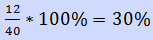Question 49
Which of the following is the BEST estimate of (3995)(102)?

 A 450,000 B 400,000 C 350,000 D 300,000 E 250,000
Question 49 Explanation:
The correct answer is (B). The number 3995 is close to 4000, and the number 102 is close to 100. The estimated product can then be written as (4000)(100) = 400,000. (Note that the actual product is 407,490.)
 Question 50
Jodi buys nine pens at \$1.10 per pen. Which of the following represents the change she would receive if she purchased the pens using a \$20 bill?

 A \$20 – (9)(\$1.10) B (\$20 – 9)(\$1.10) C \$20 + (9)(\$1.10) D (\$20 – \$1.10)(9) E \$20 – 9 – \$1.10
Question 50 Explanation:
The correct answer is (A). The cost of the nine pens is (9)(\$1.10). Since Jodi paid with a \$20 bill, her change will be the difference between \$20 and the cost of the nine pens. Thus, her change is \$20 – (9)(\$1.10).
 Question 51
Which of the following tables does NOT represent a function?

 A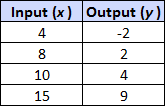BC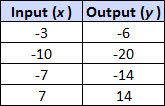DEQuestion 51 Explanation:
The correct answer is (D). By definition, a function cannot show two or more of the same outputs for any single input. This can be remembered as “a function must pass the vertical line test; the curve of the function cannot have two points lying along any vertical line drawn through the function.” Stated otherwise, each input value must have only one output value. Answer choice (D) is not a function because when x = 4, y can equal 15 or 20.
Once you are finished, click the button below. Any items you have not completed will be marked incorrect.
There are 51 questions to complete.
 ← List →
Shaded items are complete.
 1 2 3 4 5 6 7 8 9 10 11 12 13 14 15 16 17 18 19 20 21 22 23 24 25 26 27 28 29 30 31 32 33 34 35 36 37 38 39 40 41 42 43 44 45 46 47 48 49 50 51 End